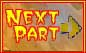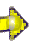Another thing scientists measure in waves is the wave's amplitude or height. What exactly do we mean by a wave's "amplitude". That is, how do you measure the height or amplitude of a wave? Look at these diagrams and see if you can figure out a good definition for amplitude.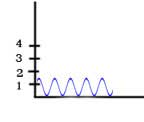The Amplitude is 0.7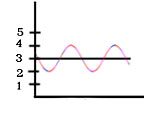The Amplitude is 1Select the definition below that you think best fits the amplitudes shown above and click here if you want to know whether you've got the one scientists use!

• a measurement from the lowest point that the wave hits to the highest point the wave hits.
• a measurement of the top half of the wave.
• a measurement of the distance between two nearest peaks or two nearest troughs.
• a measurement of the bottom half of the wave.

Try this out!

Test yourself on this diagram
The amplitude of this wave is...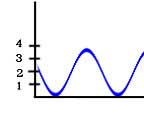In astronomy, amplitude of a light's wave is important because it tells you about the intensity or brightness of the light relative to other light waves of the same wavelength. It's a measure of how much energy the wave carries.So, if the light from Star A is brighter than the same type of light from Star B, you know that the light you see from Star A has the larger amplitude. Its very important to note that while amplitude gives you information about the intensity of the light, it is the wavelength, not the amplitude, that tells you what kind of light it is.

 Remember, Wavelength tells you the type of light And, Amplitude tells you about the intensity of the light

So, now you're ready to go ahead with the rest of the light tour!